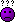# Simple question on percentages!

#### Morthoseth

Associate
My god, the years of MMORPG's and Online Gaming really have took their toll on my basic knowledge!

Right, you will be shocked at how simple this question is - but how do you go about adding up the chance for one thing to occur then for another to occur straight after?

Say 20% chance to win a certain game, and then a 20% chance to win the game after - a very simple example, but how do i work out the percentage to work out the chance of winning both games (the working out?)

My brain just wont work this morning! To think i was considering doing computer science next year! Lol!

#### Rotty

Don
long time since I did maths but surely it is 20% of 20%

so 4%

Associate
..

Last edited:

#### AGD

Soldato
You use the AND rule - the chance of A AND B is probability of A multiplied by probability of B.

In your case it would be 1/5 * 1/5 = 1/25 or 4%.

#### Rotty

Don
You multiply the chance of each happening together AFAIK. So using you're example, a 1/5 chance of each happening is 1/5*1/5 = 2/25 = 4%

except that the answer is 1/25 not 2/25, isn't it ?

#### AGD

Soldato
1/5*1/5 = 2/25 = 4%

FAIL.#### Morthoseth

Associate
OP
Or not!
Although maybe i was along the right lines i just copied his typo, 20 and 25% = 1/5 * 1/4 = 1/20 = 5%?

#### MarkDavis

Soldato
You multiply the chance of each happening together AFAIK. So using you're example, a 1/5 chance of each happening is 1/5*1/5 = 2/25 = 4%

LOL!

[Edit: sorry, originally quoted the bibalavegas post instead of F0rb's!]

Last edited:

#### Nitefly

Man of Honour

...#### AGD

SoldatoThere is a lot of bad maths in this thread but I don't think any of it was mine!

#### MarkDavis

Soldato
Just to complicate matters, if you're doing this properly, its not (the chance of A occurring) x (the chance of B occurring) that is the key here. The key is actually whether you're correctly calculating the probabilities of A or B occurring. Take a simple example. If the chance of you winning the first game (say, event A) is 20% or 1/4, then you play the game again (assuming the game is the same), then B should be less than 20% or less than 1/4 because of the increase in familiarity, experience, possibly skill etc.

#### mac1st3

Soldato
*whoooshing noises are heard above Mac's head* >.>

#### Morthoseth

Associate
OP
Thanks for the help guys, knew i could count on you.

Cant believe I'd forgotten how to do it though, quite shameful really! Lol!

#### fez

Soldato
Thanks for the help guys, knew i could count on you.

Cant believe I'd forgotten how to do it though, quite shameful really! Lol!

Dont sweat it man, there were quite a few people on here who didnt realise they couldnt do multiplication, at least you are enlightened.

#### QlimaxUK

Soldato
4%

How to work out:
20% = 1/5
20 and 20 = 1/5*1/5
1/5*1/5 = 1/25
---------------------------------------------
100 / 25 = 4 so 4/100 = 4%

understand?#### Nitefly

Man of Honour
Thanks for the help guys, knew i could count on you.

Cant believe I'd forgotten how to do it though, quite shameful really! Lol!

I wouldn't worry, we all forget something simple once in awhile!

#### mattheman

Permabanned
You multiply the chance of each happening together AFAIK. So using you're example, a 1/5 chance of each happening is 1/5*1/5 = 2/25 = 4%

Thats just off the top of my head, might be totally wrong. I could work it out properly with all probabilities, but that would take a while1*1=1

#### Moredhel

Soldato
Erm, if the chances of winning a game are 20% and you play the exact same game again, the chances of winning that are also 20%... The chance doesn't change just because you've done it in sequence.

It's the same as randomly pulling cards out of a deck and getting all 4 aces. The chances of you getting an ace actually go up each time as there's less cards in the set.

#### PMKeates

Soldato
Erm, if the chances of winning a game are 20% and you play the exact same game again, the chances of winning that are also 20%... The chance doesn't change just because you've done it in sequence.

It's the same as randomly pulling cards out of a deck and getting all 4 aces. The chances of you getting an ace actually go up each time as there's less cards in the set.
As an independent event, yes. Otherwise, no. On your principal the probability of a roulette wheel being red for 1,000 goes is the same as it being 500 black and 500 red.

#### The Halk

Soldato
Just multiply the percentages. 20% is 0.2. So 0.2*0.2=0.04 which is 4%